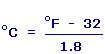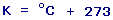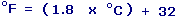# Temperature Conversion Calculator

Calculate and convert the temperature into celsius, faherenheit & kelvin from any given unit using formulas given below.

 Enter Value: Value In: Celsius Faherenheit Kelvin

 Celsius: faherenheit: Kelvin:

Fahrenheit to Celsius:## Method to find higher temperature if temperature units are different

To compare temperature all the temperature should have same unit. For example:

Find the higher temperature out of two given temeparature 39oC and 49oF.

Now we should have same units. means lets convert 49oF in celsius.

Using calculator 49oF = 9.4452oC

Now we can compare both temperature in oC and we can say

39oC is higher than 49oF.